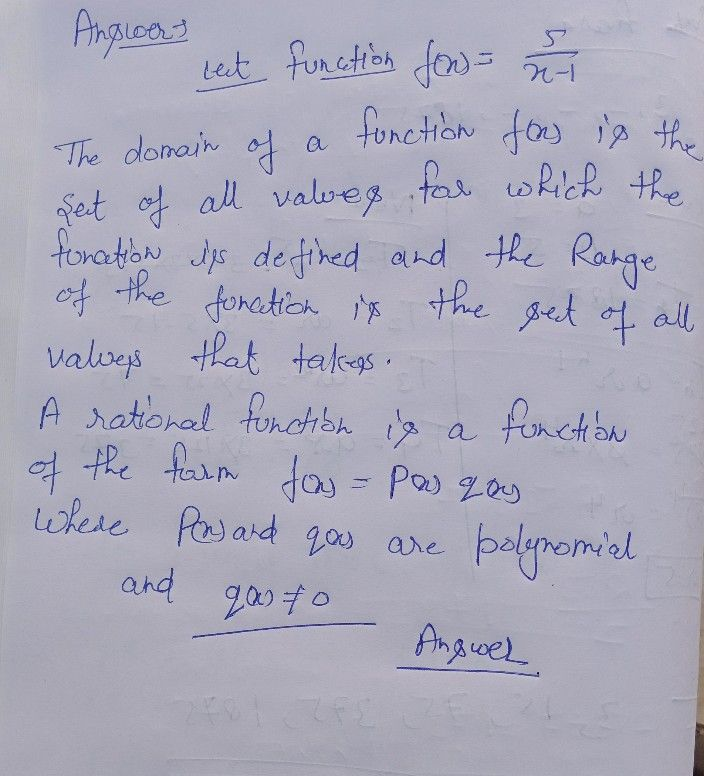Symbol
ProblemThis module is about the $1o11\right)tai1$ and $11\right)g0$ of a rational function. It laid down the basic $conCcpts$ of domain and range $1111$ showed how to determine them in a rational function. From this module, you learned that a function is a simple rule of correspondence between two variables xand y. The x variable is considered the input which is also called the independent variable while the y variable is the output which is also called the dependent variable. It is a basic notion that for every value there corresponds a value in y. This set of values in x is the domain while the soef t ox f values in $yis$ the range of a rational function. $\left(018$ you try to summarize on your own by $1$ in the blanks $T0$ determine the domain and range in rational functions, the denominator to $a\pi$ solve for the variable x The $0bcc1x$ is that it must have denominator. The value that would make it zero is the value that woukd included in the domain. To find the range, solve the equation for x in terms $2$ $x$ in $Aain$ it must have $1011$ $1$ denominator. The value that would make $\bar{x}$ equal to $x$ is the value that would not be inchaded in the range.
10th-13th grade
Other
Search count: 120
SolutionQanda teacher - henrysee